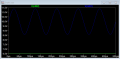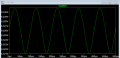# Voltage regulating with a Zener Diode

#### uhf737

Joined Dec 9, 2017
14
Hi.
I have a problem with this homework, I have to calculate the peak voltage of the output after stabilizing with a zener diode.

My Input Voltage is Vin = 5V + 0.7V*sin (2pi*f*t) with f=10kHz

My circuit is a simple regulator with no load resistor. The series resistance is 82 Ohms, Zener voltage is 3V, max. current through the diode is 33.3mA. How can I calculate the peak output ? My problem is the offset created by 5V, wouldnt the Zener voltage be reachey immidiately with this configuration ?

#### crutschow

Joined Mar 14, 2008
30,117

#### uhf737

Joined Dec 9, 2017
14

#### WBahn

Joined Mar 31, 2012
26,489

#### uhf737

Joined Dec 9, 2017
14
The OP has all info I have. Is it not possible to calculate the output peak with that information ? Let's say my sinewave has no offset. That would mean the peak is at 3V wouldnt it ? It would look almost like a rectangle wave for the positive wave, and would be ~0 for the negative ones.

#### WBahn

Joined Mar 31, 2012
26,489
The OP has all info I have. Is it not possible to calculate the output peak with that information ? Let's say my sinewave has no offset. That would mean the peak is at 3V wouldnt it ? It would look almost like a rectangle wave for the positive wave, and would be ~0 for the negative ones.
Depending on what you mean by "almost" and "~0" you may have the right idea. A sketch would go along way to confirming or refuting that.

What would the waveform look like if the zener were removed?

That's what it will look like during the times that the zener isn't conducting at all.'

No figure out were the zener is forward conducting and where it is reverse conducting and replace it will the appropriate model for those regions.

What would the waveform look like if the amplitude of the sine wave was 4 V?

#### MrChips

Joined Oct 2, 2009
26,505
If you can assume that the diode is an ideal zener diode with 3V zener voltage then isn't the max output voltage 3V?

#### uhf737

Joined Dec 9, 2017
14
If you can assume that the diode is an ideal zener diode with 3V zener voltage then isn't the max output voltage 3V?
Yes but the 5V offset is confusing me. Is the peak output still 3V if it has an offset ?

Depending on what you mean by "almost" and "~0" you may have the right idea. A sketch would go along way to confirming or refuting that.

What would the waveform look like if the zener were removed?

That's what it will look like during the times that the zener isn't conducting at all.'

No figure out were the zener is forward conducting and where it is reverse conducting and replace it will the appropriate model for those regions.

What would the waveform look like if the amplitude of the sine wave was 4 V?
Assuming there is no offset, the output would be a regular sinewave till the zener voltage is reached, then it would be constant till the input drops around -0.7V because it acts like a regular diode if forward biased.

How does this work with my offset ?

#### WBahn

Joined Mar 31, 2012
26,489
Yes but the 5V offset is confusing me. Is the peak output still 3V if it has an offset ?

Assuming there is no offset, the output would be a regular sinewave till the zener voltage is reached, then it would be constant till the input drops around -0.7V because it acts like a regular diode if forward biased.

How does this work with my offset ?
Doesn't it work the exact same way?

The zener diode doesn't know that part of the voltage is a sine wave and part is a DC offset. It sees a voltage. Voltages above the zener voltage are clamped at the zener voltage. Voltages below -0.7 V are clamped because it's like a regular voltage.

#### uhf737

Joined Dec 9, 2017
14
Doesn't it work the exact same way?

The zener diode doesn't know that part of the voltage is a sine wave and part is a DC offset. It sees a voltage. Voltages above the zener voltage are clamped at the zener voltage. Voltages below -0.7 V are clamped because it's like a regular voltage.
That would mean the peak is always at 3V (in my case ) ?

I am asking because part of the homework was calculating the remaining AC voltage peak and the DC voltage offset at the output but what is there to calculate if this is the case ?

#### uhf737

Joined Dec 9, 2017
14
Scratch that, since the offset is causing the input voltage to be >Vout the zener diode is always clamping. I made a simulation (slightly different parameters since i couldnt find a zener diode with 3V breakdown voltage) with ltspice and got this output wave. Green is output, blue is input.Zener breakdown is 6.2V, I assume my series resitance is poorly chosen.this is what it looks like zoomed inNo idea why its fluctuating, does it have something to do with my series resistance ?

#### MrChips

Joined Oct 2, 2009
26,505
Yes, it is because of internal resistance of the diode.
It is fluctuating because it is a real diode with real characteristics and not an ideal diode.
An ideal diode has infinite resistance below the zener voltage and zero resistance above the zener voltage.

#### WBahn

Joined Mar 31, 2012
26,489
Scratch that, since the offset is causing the input voltage to be >Vout the zener diode is always clamping. I made a simulation (slightly different parameters since i couldnt find a zener diode with 3V breakdown voltage) with ltspice and got this output wave. Green is output, blue is input.Zener breakdown is 6.2V, I assume my series resitance is poorly chosen.

View attachment 141445

this is what it looks like zoomed in

View attachment 141446

No idea why its fluctuating, does it have something to do with my series resistance ?
Not so much the series resistance you have in the circuit, but the fact that the voltage across the zener diode is not perfectly flat as you change the current through it. The more current you pass through it, the more the voltage across it will be. It is a shallow slope (meaning low effective resistance) but it is included in the simulation model for the diode.

The same behavior is true of real diodes when forward biased. The more the current, the more the voltage. As a rough rule of thumb, if you increase the current through a silicon diode near room temperature by a factor of ten (a one decade increase in current), the forward voltage drop increases by about 20 mV to 30 mV. For the base-emitter junction of a BJT it's about 60 mV/decade.

#### uhf737

Joined Dec 9, 2017
14
Thanks for the help.

I understand whats happening in my circuit but that doesnt really help me with the task. I have to calculate the remaining AC voltage peak and the DC voltage at the output. I have a small section of the datasheet but the values are only for specific currents.

Vz @ 5mA = 3V
Zzt @ 5mA = 89 Ohms

Zzk@ 1mA = 564 Ohms (I assume this is the minimum current needed for the zener diode)

Ir@1V = 9uA (No idea what this stands for)

my series resistance is Rs = 82 Ohms, Vin = 5V+0.7sin (wt) f=10kHz, Uz=3V

This is all the info I have to solve it.

#### WBahn

Joined Mar 31, 2012
26,489

Zzk is the effective series resistance of the zener at the knee voltage.

Zzt is the total effective series resistance of the zener at that current.

Given only two data points, you have to make some kind of estimate of what the resistance is at other currents. The simplest is to assume that the effective series resistance from 5 mA up to the ~35 mA is roughly the same at 89 Ω. State that assumption and then work the problem with that assumption.

Ir is the reverse current which means that when the reverse voltage is 1 V, the current through the diode is about 9 uA.

#### MrAl

Joined Jun 17, 2014
9,174
Thanks for the help.

I understand whats happening in my circuit but that doesnt really help me with the task. I have to calculate the remaining AC voltage peak and the DC voltage at the output. I have a small section of the datasheet but the values are only for specific currents.

Vz @ 5mA = 3V
Zzt @ 5mA = 89 Ohms

Zzk@ 1mA = 564 Ohms (I assume this is the minimum current needed for the zener diode)

Ir@1V = 9uA (No idea what this stands for)

my series resistance is Rs = 82 Ohms, Vin = 5V+0.7sin (wt) f=10kHz, Uz=3V

This is all the info I have to solve it.

Hello,

Wow, that's a lot more than you originally gave. That could change a lot here.

But also you need to check the max current through the diode. IF the diode is rated for 33ma as you say in one post, then with your signal of 5v plus some sine, you have to make sure that the current never goes above that. Alternately, you would compute the power in the diode and make sure it never goes above the power that the diode is rated for (if you have that information that is). Computing the power would be the more practical thing to do to make sure the diode does not burn out. If the power is too high, you either need to use a higher power diode or increase the 82 Ohm resistor so that the power is not too high, if that is allowed that is. If you can not do that then the circuit will burn out so it's not yet ready for real time#### Bordodynov

Joined May 20, 2015
2,995

#### Bordodynov

Joined May 20, 2015
2,995
What I showed is an approximation to reality, but not reality. For a good match of reality, it is necessary to measure real characteristics (voltage as a function of current) in a full range of currents. I adjusted the characteristics only for a range of 1 mA - 5 mA. This may not be enough!

#### uhf737

Joined Dec 9, 2017
14
Thanks for replies, I really appreciate it.

I tried solving it without simulations and this is what I got :

Datasheet says max. dissipation is 0.1W=Pmax

Series resistance of the diode is Zzt = 89 Ohm (I will assume its nearly the same from 5mA-35mA) Vzt@Izt = 3V, Izt=5mA

Equivalent circuit of a diode is rz and Uz0 in series.

That means what I am looking for is Vzmax = Vz0+Izmax*rz

Vz0 is defined as Vz0 = Vzt@Izt - Izt*rz = 3V-5mA*89Ohms = 2.55V

Pzmax = Vzmax*Izmax = (Vz0+Izmax*rz)*Izmax

Solving this quadratic equation gives me Izmax = 22.1mA and Vzmax = 4.53V which is the peak I was looking for ?

I would have to adjust the series resistance to R = (5.7V-4.53V)/22.1mA = 53.2 Ohms though if what I calculated was right